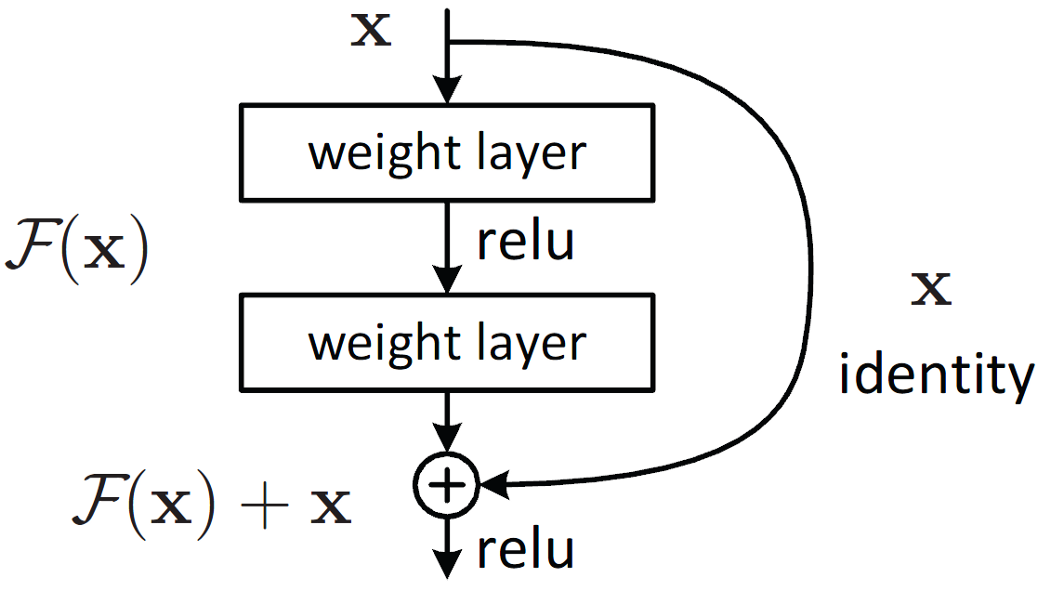# 如何使用PyTorch简易搭建残差网络# 残差架构\$\$
y=F(x)+x
\$\$
F(x)x的维度不匹配时，我们可以简单地在跳跃连接的过程中进行线性投影来改变x的维度。

## 计算环境

### 库

``````"""
The following is an import of PyTorch libraries.
"""
import torch
import torch.nn as nn
import torch.nn.functional as F
import torchvision
from torchvision import datasets, transforms
from torchvision.utils import save_image
import matplotlib.pyplot as plt
import numpy as np
import random
``````

### 硬件要求

``````"""
Determine if any GPUs are available
"""
device = torch.device('cuda' if torch.cuda.is_available() else 'cpu')
``````

## 搭建残差块

``````"""
Define an nn.Module class for a simple residual block with equal dimensions
"""
class ResBlock(nn.Module):

"""
Initialize a residual block with two convolutions followed by batchnorm layers
"""
def __init__(self, in_size:int, hidden_size:int, out_size:int):
super().__init__()
self.conv1 = nn.Conv2d(in_size, hidden_size, 3, padding=1)
self.conv2 = nn.Conv2d(hidden_size, out_size, 3, padding=1)
self.batchnorm1 = nn.BatchNorm2d(hidden_size)
self.batchnorm2 = nn.BatchNorm2d(out_size)

def convblock(self, x):
x = F.relu(self.batchnorm1(self.conv1(x)))
x = F.relu(self.batchnorm2(self.conv2(x)))
return x

"""
Combine output with the original input
"""
def forward(self, x): return x + self.convblock(x) # skip connection
``````

## 使用既有的ResNet模型

``````"""
Creates a pretrained on ImageNet Resnet34
"""
resnet = torchvision.models.resnet34(pretrained=True)
``````